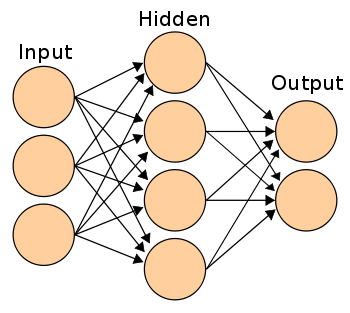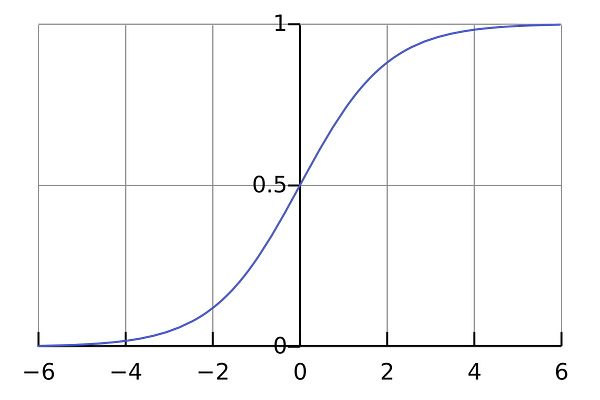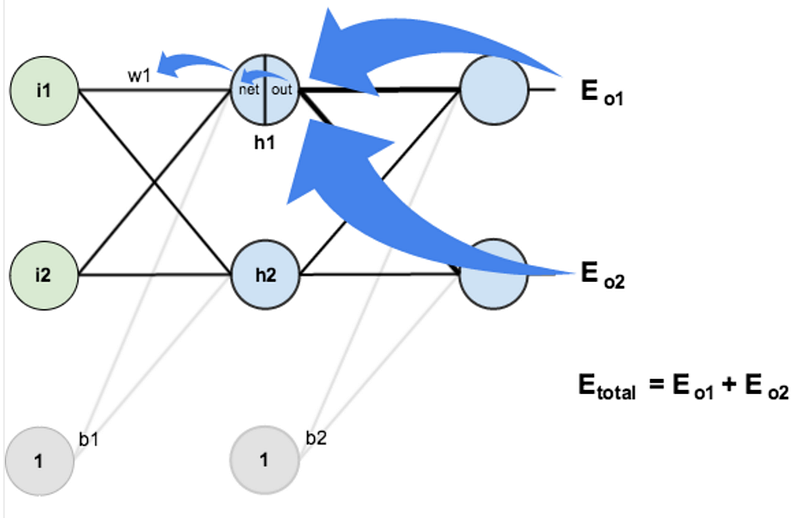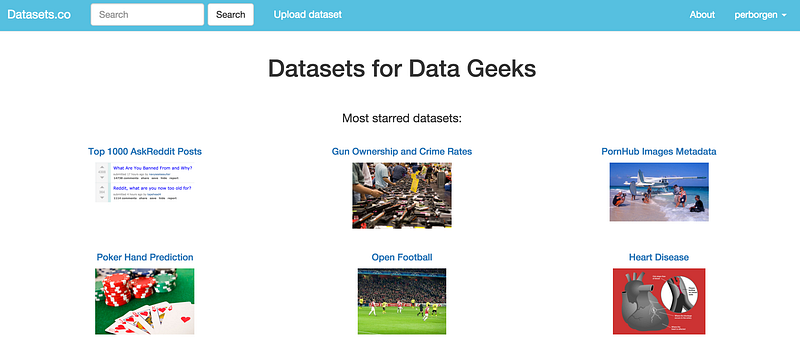4.2. Algorithmes

# Apprendre à coder un réseau de neurones

This is the second post in a series of me trying to learn something new over a short period of time. The first time consisted of learning how to do machine learning in a week.

This time I’ve tried to learn neural networks. While I didn’t manage to do it within a week, due to various reasons, I did get a basic understanding of it throughout the summer and autumn of 2015.

By basic understanding, I mean that I finally know how to code simple neural networks from scratch on my own.

In this post, I’ll give a few explanations and guide you to the resources I’ve used, in case you’re interested in doing this yourself.

#### Step 1: Neurons and forward propagation

So what is a neural network? Let’s wait with the network part and start off with one single neuron.

A neuron is like a function; it takes a few inputs and calculates an output.

The circle below illustrates an artificial neuron. Its input is 5 and its output is 1. The input is the sum of the three synapses connecting to the neuron (the three arrows at the left).

At the far left we see two input values plus a bias value. The input values are 1 and 0 (the green numbers), while the bias holds a value of -2 (the brown number).

The inputs here might be numerical representations of two different features. If we’re building a spam filter, it could be wether or not the email contains more than one CAPITALIZED WORD and wether or not it contains the word ‘viagra’.

The two inputs are then multiplied by their so called weights, which are 7 and 3 (the blue numbers).

Finally we add it up with the bias and end up with a number, in this case: 5 (the red number). This is the input for our artificial neuron.

The neuron then performs some kind of computation on this number — in our case the Sigmoid function, and then spits out an output. This happens to be 1, as Sigmoid of 5 equals to 1, if we round the number up (more info on the Sigmoid function follows later).

If this was a spam filter, the fact that we’re outputting 1 (as opposed to 0) probably means that the neuron has labeled the text as ‘spam’.A neural network illustration from Wikipedia.

If you connect a network of these neurons together, you have a neural network, which propagates forward — from input output, via neurons which are connected to each other through synapses, like on the image to the left.

I can strongly recommend theWelch Labs videos on YouTube for getting a better intuitive explanation of this process.

#### Step 2: Understanding the Sigmoid function

After you’ve seen the Welch Labs videos, its a good idea to spend some time watching Week 4 of the Coursera’s Machine Learning course, which covers neural networks, as it’ll give you more intuition of how they work.

The course is fairly mathematical, and its based around Octave, while I prefer Python. Because of this, I did not do the programming exercises. Instead, I used the videos to help me understand what I needed to learn.

The first thing I realized I needed to investigate further was the Sigmoid function, as this seemed to be a critical part of many neural networks. I knew a little bit about the function, as it was also covered in Week 3 of the same course. So I went back and watched these videos again.The Sigmoid function simply maps your value (along the horizontal axis) to a value between 0 and 1.

But watching videos won’t get you all the way. To really understand it, I felt I needed to code it from the ground up.

So I started to code a logistic regression algorithm from scratch (which happened to use the Sigmoid function).

It took a whole day, and it’s probably not a very good implementation of logistic regression. But that doesn’t matter, as I finally understood how it works. Check the code here.

You don’t need to perform this entire exercise yourself, as it requires some knowledge about and cost functions and gradient descent, which you might not have at this point.

But make sure you understand how the Sigmoid function works.

#### Step 3: Understanding backpropagation

Understanding how a neural network works from input to output isn’t that difficult to understand, at least conceptually.

More difficult though, is understanding how the neural network actually learns from looking at a set of data samples.

The concept is called backpropagation.

This essentially means that you look at how wrong the network guessed, and then adjust the networks weights accordingly.

The weights were the blue numbers on our neuron in the beginning of the article.

This process happens backwards, because you start at the end of the network (observe how wrong the networks ‘guess’ is), and then move backwards through the network, while adjusting the weights on the way, until you finally reach the inputs.

To calculate this by hand requires some calculus, as it involves getting some derivatives of the networks’ weights. The Kahn Academy calculus courses seems like a good way to start, though I haven’t used them myself, as I took calculus on university.

Note: there are a lot of libraries that calculates the derivatives for you, so if you’d like to start coding neural networks before completely understanding the math, you’re fully able to do this as well.Screehshot from Matt Mazurs tutorial on backpropagation.

The three best sources I found for understanding backpropagation are these:

You should definitely code along while you’re reading the articles, especially the two first ones. It’ll give you some sample code to look back at when you’re confused in the future.

Plus, I can’t really emphasize this enough:

You don’t learn much by reading about neural nets, you need to practice it to make the knowledge stick.

The third article is also fantastic, but I’ve used this more as a wiki than a plain tutorial, as it’s actually an entire book. It contains thorough explanations all the important concepts in neural networks.

These articles will also help you understand important concepts as cost functions and gradient descent, which play equally important roles in neural networks.

#### Step 3: Coding your own neural networks

In some articles and tutorials you’ll actually end up coding small neural networks. As soon as you’re comfortable with that, I recommend you to go all in on this strategy. It’s both fun and an extremely effective way of learning.

One of the articles I also learned a lot from was A Neural Network in 11 Lines Of Python by IAmTrask. It contains an extraordinary amount of compressed knowledge and concepts in just 11 lines.

After you’ve coded along with this example, you should do as the article states at the bottom, which is to implement it once again without looking at the tutorial. This forces you to really understand the concepts, and will likely reveal holes in your knowledge, which isn’t fun. However, when you finally manage it, you’ll feel like you’ve just acquired a new superpower.

A little side note: When doing exercises I was often confused by the vectorized implementations some tutorials use, as it requires a little bit of linear algebra to understand. Once again, I turned myself back to the Coursera ML course, as Week 1 contains a full section of linear algebra review. This helps you to understand how matrixes and vectors are multiplied in the networks.

When you’ve done this, you can continue with this Wild ML tutorial, by Denny Britz, which guides you through a little more robust neural network.

At this point, you could either try and code your own neural network from scratch or start playing around with some of the networks you have coded up already. It’s great fun to find a dataset that interests you and try to make some predictions with your neural nets.

To get a hold of a dataset, just visit my side project Datasets.co (← shameless self promotion) and find one you like.Visit Datasets.co to get hold of a dataset

Anyway, the point is that you’re now better off experimenting with stuff that interests you rather than following my advices.

Personally, I’m currently learning how to use Python libraries that makes it easier to code up neural networks, like Theano, Lasagne and nolearn. I’m using this to do challenges on Kaggle, which is both great fun and great learning.

Good luck!

And don’t forget to press the heart button if you liked the article 🙂

Thanks for reading! My name is Per Borgen and I mostly write about learning new stuff.

Feel free to reach out to me if you have and questions or would like to connect, either through Twitter, GitHub or just plain old email:perhborgen@gmail.com.